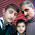## Friday, November 15, 2013

### Diamond With 3 Do While Loops

Code:

```// http://welearncpp.blogspot.com
#include<iostream>
using namespace std;

void main()
{
int row,space,star=1;
cout << "Enter an odd number :";
cin >> row;
if((row%2)==0)
row--;
space=row;
int a=1;
do
{
int b=1;
do
{
cout << " ";
b++;
} while(b<=space);

int c=1;
do
{
cout << "*";
c++;
} while (c<=star);
if(a<=row/2)
{
space--;
star+=2;
}
else
{
space++;
star-=2;
}
cout<<endl;
a++;
} while(a<=row);
}```

#### 1 comment:

1.Nice job with deep research and Thank you very much for sharing your unique review with us and Stay blessed